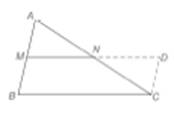Chapter 4.2, Problem 43EElementary Geometry For College St...

7th Edition
Alexander + 2 others
ISBN: 9781337614085

Solutions

Chapter
SectionElementary Geometry For College St...

7th Edition
Alexander + 2 others
ISBN: 9781337614085
Textbook Problem

Prove that the segment that joins the midpoints of two sides of a triangle has a length equal to one-half the length of the third side.(HINT: In the drawing, M N ¯ is extended to D , a point on C D ¯ . Also, C D ¯ is a parallel to A B ¯ .)To determine

To prove:

The segment that joins the midpoints of two sides of a triangle has a length equal to one-half the length of the third side.

Explanation

Formal proof:

Statement:

The segment that joins the midpoints of two sides of a triangle has a length equal to one-half the length of the third side.

Drawing:

Given:

ABC is a triangle with M and N as the midpoint of the sides AB and AC respectively.

ANCN

AMBM

Prove:

MN=12BC

 PROOF Statement Reasons 1. AM¯≅BM¯AN¯≅CN¯ 1. Given 2. Produce MN to D to meet CD which is parallel to AB. 2. Construct a parallelogram BCDM 3

Still sussing out bartleby?

Check out a sample textbook solution.

See a sample solution

The Solution to Your Study Problems

Bartleby provides explanations to thousands of textbook problems written by our experts, many with advanced degrees!

Get Started

Find more solutions based on key concepts